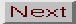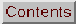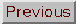11.2. Orbits in a Barred Galaxy

Knowledge of the stellar orbits in a barred galaxy can help us to understand the dynamical properties of bars, their extension, and pattern velocity. It is also fundamental to understanding resonances, the behavior of the gas component, and therefore to explaining the existence of rings.

Bars are three-dimensional components, and they can be modeled as triaxial ellipsoids. Although the disks of spiral galaxies are very thin components, with axis ratios around 10, bars can be thicker because of vertical resonances (e.g. Combes et al. 1990b), and reveal box or peanut shapes when seen edge-on. In a first approach, we will describe the stellar orbits as if they were confined in the galaxy plane. This much simpler approach gives already most of the characteristics of bar dynamics and is sufficient to understand the gas behavior, since the gas disk is much thinner.

First, let us recall the characteristics of orbits in an axisymmetric potential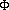(r) in the plane z = 0. A circular orbit has an angular velocity2 = 1 / r d/ dr. In linearizing the potential in the neighborhood of a circular orbit, the motion of any particle can be expressed in first order by an epicyclic oscillation, of frequency,2 = d2/ dr 2 + 32 = r d2 / dr + 42 6

The general orbit is therefore the combination of a circle and an epicycle, or a rosette, since there is no rational relation between the two periods.

The bar creates a bisymmetric gravitational potential, with a predominant Fourier component m = 2, which rotates in the galaxy with the pattern speedb. To be left with a potential independent of time, where the energy of particles is conserved, we must consider the orbits in the rotating frame. The equivalent potential in this frame is then:eq =(r,) - 1/ 2b2 r2 7

in cylindrical coordinates (r,, z), for z = 0.

The energy of a particle (per unit mass) in this frame is

EJ = 1/ 2 v2 +- 1/ 2b2 r2. 8

It is an integral called the Jacobi integral. It is expressed as a function of the energy in the fixed frame E, as EJ = E -b Lz , where Lz is the angular momentum, which is not an integral, since the potential is non-axisymmetric.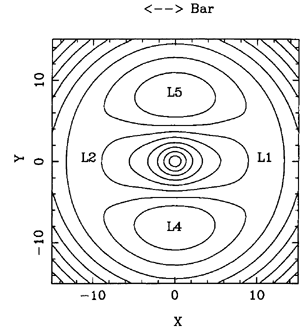The equipotentials in the rotating frame are given in Figure 58. There exist 5 stationary points, known as Lagrangian points, L1 to L5, where the derivative ofeq vanishes. L3 is the central minimum, L4 and L5 are maxima, and L1 and L2 are saddle points. Only L4 and L5 are stable, i.e., a particle can oscillate around them with the epicyclic frequency. The four points, L1, L2, L4, and L5 correspond to the corotation resonance with the bar. Although they are not located at the same galactocentric distance, they delineate an annular zone, called the corotation zone.

In the rotating frame, the effective angular velocity of a particle is' =-b. There exists then regions in the galaxy where' =/ m, i.e. where the epicyclic orbits close themselves after m lobes. The corresponding stars are aligned with the perturbation and closely follow it; they interact with it always with the same sign, and resonate with it. These zones are the Lindblad resonances, sketched with dashed circles in Figure 59a. According to the relative values ofandin a realistic disk galaxy (Figure 59b), and because the bar is a bisymmetric perturbation, the most important resonances are those for m = 2. An obvious resonance is corotation, for which=b (' = 0).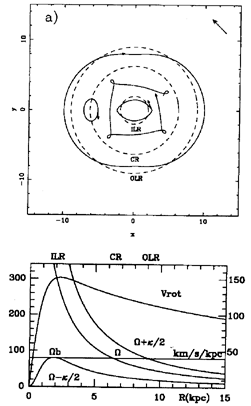Periodic Orbits

Periodic orbits in the bar rotating frame are orbits that close on themselves after one or more turns. Periodic orbits are the building blocks which determine the stellar distribution function, since they define families of trapped orbits around them. Trapped orbits are non-periodic, but oscillate about one periodic orbit, with a similar shape. The various families are best identified in surface of section diagrams, first used by Poincaré as early as 1899. This method consists of representing orbits of the 4-D phase-space (x, y, xdot, ydot) only by their intersection points with any plane of phase space, for instance xdot = 0, and projecting these points in a 2-D space (for instance (y, ydot)). Periodic orbits appear then as single points, while trapped orbits are represented by invariant curves. The fact that points do not spread in space all over a given region, but follow an invariant curve, is the consequence of the existence of another integral besides Jacobi's. There can, however, exist irregular orbits, for which the intersection points are spread all over a sea in a stochastic manner. These ergodic orbits occur essentially when the potential presents strong asymmetries. For realistic galaxy potentials, ergodic orbits concern mainly regions outside corotation.

The periodic orbits are numerous (see the recent review by Contopoulos & Grosbøl 1989), and we will describe here the most important ones for the bar support, and for the ring formation mechanism:

1. the x1 family (see Figure 60) is the main family supporting the bar. Orbits are elongated parallel to the bar, within corotation. They can look like simple ellipses, and with energy increasing, they can form a cusp, and even two loops at the extremities.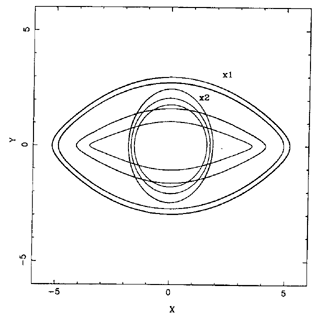2. the x2 family exists only between the two inner Lindblad resonances (ILR), when they exist. They are more round, and elongated perpendicular to the bar. In the same category of 2 / 1 orbits (which close after one turn and two epicycles), there are also the x4 retrograde orbits, and the x3 unstable orbits, but with less impact for galaxies. The presence or not of nuclear rings appears to be related to the existence of an ILR, as we have discussed in sections 9.7 and 9.8, and which will be developed later. Even when there exist two ILR's in the axisymmetric sense, the existence of the x2 family is not certain. When the bar is strong enough, the x2 orbits disappear. The bar strength necessary to eliminate the x2 family depends on the pattern speedb: the lower this speed, the stronger the bar must be.

3. Outside corotation, the 2 / 1 orbits that are run in the retrograde sense in the rotating frame are perpendicular to the bar inside the outer Lindblad resonance (OLR), and parallel to the bar slightly outside (Figure 61). These orbits are also discussed by Kalnajs (1991). Their shape reveals a characteristic figure-eight, that is very similar to the dimpled shape of some outer rings in barred galaxies (see section 4.1 and Figure 18). When the bar is strong, most orbits perpendicular to the bar between CR and OLR become unstable. That is why this region is often depopulated and, in real galaxies, never includes recent star formation.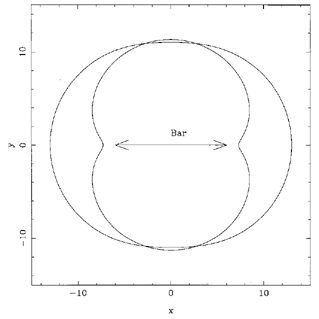4. When getting towards corotation (CR) all higher order resonances' =/ m with m3 are encountered. Spanning this region, on the inner or outer side of CR, the periodic families 6 / 1 and 4 / 1 are displayed in Figure 62. The 4 / 1 resonant family is likely to play a role in the formation of inner rings.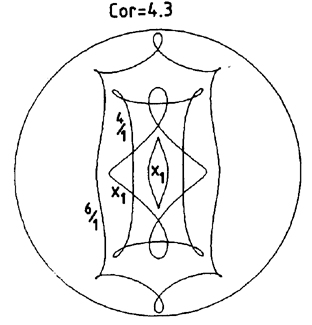5. Finally, circulating around the Lagrangian points L4 and L5, one can distinguish the banana orbits or long period orbits (LPO), and the short period orbits (SPO).

Consequences for the Bar Dynamics

The main family of orbits building the bar is the x1 family, elongated along it. We can note however that the stable direct periodic orbits have an orientation either parallel or perpendicular to the bar, and that their orientation rotates by 90° at each Lindblad resonance crossing. The x2 family, when it exists between the two ILR's, tends to weaken the bar, if the concerned region is large enough. This suggests that a self-consistent bar can barely have one ILR; this is the same conclusion reached from the swing amplification and wave reflection mechanism to form the bar: the presence of ILR's tends to destroy the bar.

Beyond corotation, the stable periodic orbits are also perpendicular to the bar, which suggests that a self-consistent bar cannot extend far beyond its own corotation. This has been verified in N-body simulations of barred galaxies (e.g., Sellwood 1981; Combes & Sanders 1981; Sparke & Sellwood 1987): the longest bars end just slightly before their corotation.

Approaching the corotation region, higher order resonances occur, and the periodic orbits become more and more complex and squarish. The observed shape of face-on early-type galaxy bars is indeed boxy, as was shown for NGC 936 by Kormendy (1983), which compares very well with N-body models (e.g. Miller & Smith 1979). This squarish shape might measure the importance of the 4 / 1 resonance, which will in turn have a strong consequence for inner rings encircling the bars.